# Latex实践：一个零Latex基础的术渣花了一天半的时间用Latex完成一篇SCI论文编辑

## 2 术渣的Latex编辑SCI论文记录：

### 2.1 编辑前：下载模版

https://www.elsevier.com/authors/author-schemas/latex-instructions  （里面包含了Latex基本讲解和相应的模版）

### 2.2 基本编辑和认知：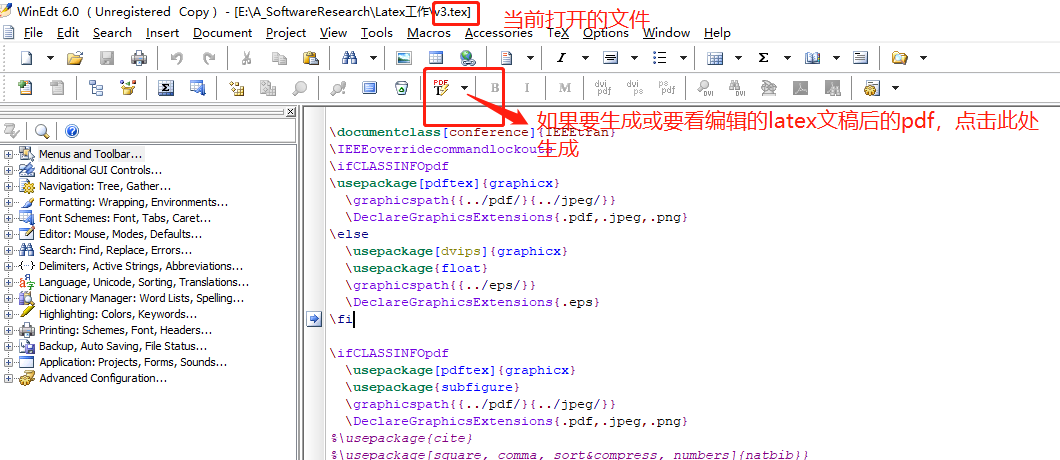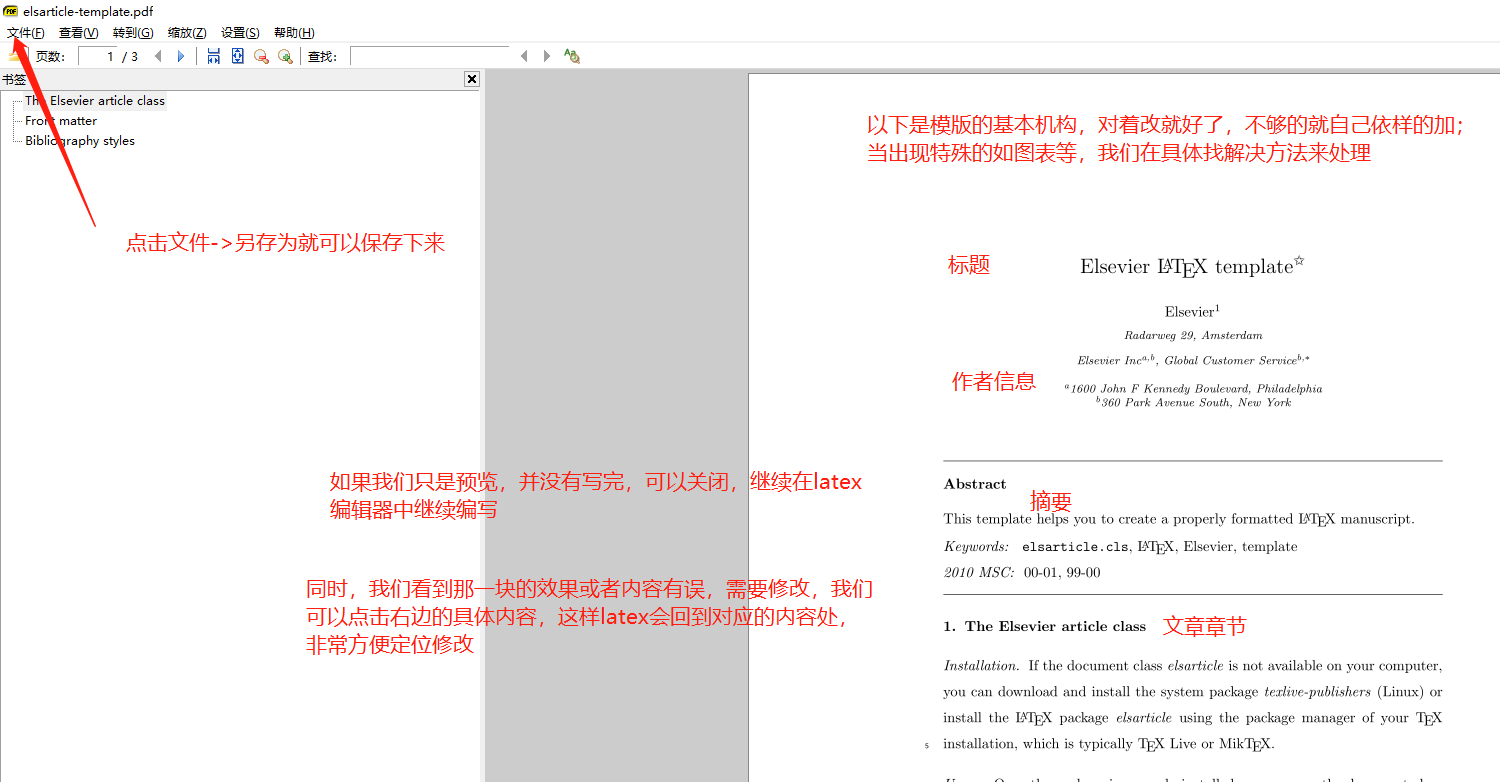\usepackage{lineno,hyperref}
\usepackage{color}
\usepackage{xcolor}
\usepackage{framed}

\title{}

\begin{abstract}
\end{abstract}

\section{Proposed approach} 一级章节

\subsection{Dense joint motion image} 二级章节 （至于往下再分，那就要百度了）

...

Tips:其实到这部分，没有什么要掌握的，对着模版改就好了，这部分应该可以完成70%左右的工作了，而剩下来的30%其实才是难啃的骨头，下面部分内容，记录我编辑论文遇到的特殊需求，可能会基本满足一篇论文特殊需求的大部分,主要有:公式的制作，表的制作，图的插入，文献的引用，文字颜色的设置（包括部分和一大块字段的设置）

### 2.3 公式的制作

(要使Mathtype的中编辑的公式可以拷贝到tex文件中，先要对MathType进行设置–>点击Preferences->Cut and Copy…，在这里面设置复制到tex格式)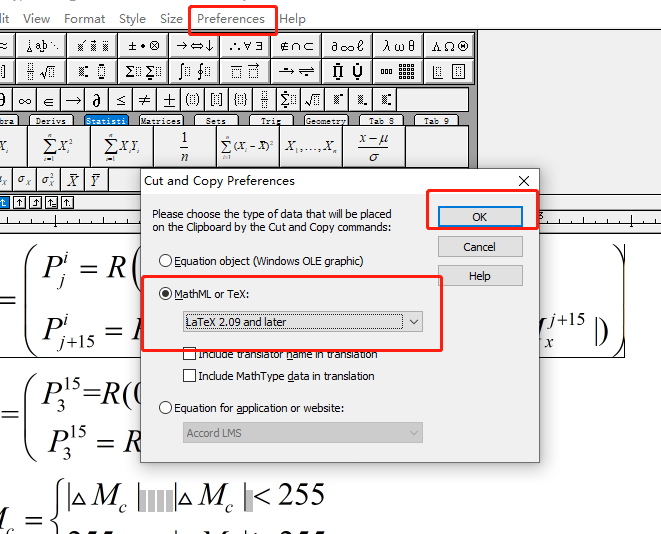2）涉及到简单的，如我只想输入一个数学字符标识α（这个输入打出来，直接写在Latex是不管用的），这种简单，或者你掌握了Latex简单式的写法后，其实可以直接用LATEX写，如α在Latex的写法是\alpha。Latex中自带了公式写法，简单的了解后可以自己写，复制的强烈建议MathType，如果不想折腾，全部用MathType写后复制到Latex也是可以的。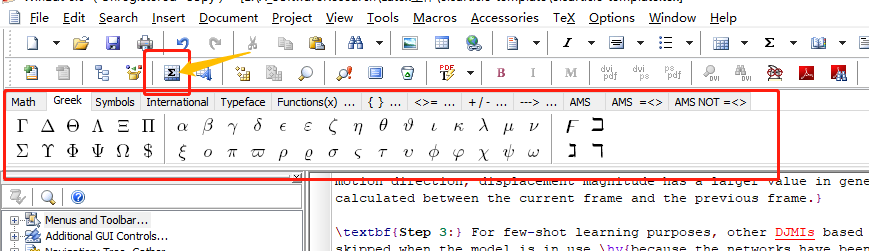3) 写完公式的文稿，尤其是稿子中包含公式的解释的，一定要仔细校稿！！！！

2）在Latex相应处直接复制，复制到LATEX后公式实际如下：(公式后面编号1...是自己累加的不需要管，写在\begin和\end里面就是表示是一个块，会另行一行)

\begin{equation}
C_j^i = \left( {\begin{array}{*{20}{l}}
{P_j^i = R\left( {M_x^j} \right),G\left( {M_y^j} \right),B\left( {M_z^j} \right)}\\
{P_{j + 15}^i = R\left( {|M_x^{j + 15}|} \right),G(|M_y^{j + 15}),B(|M_x^{j + 15}|)}
\end{array}} \right)
\end{equation}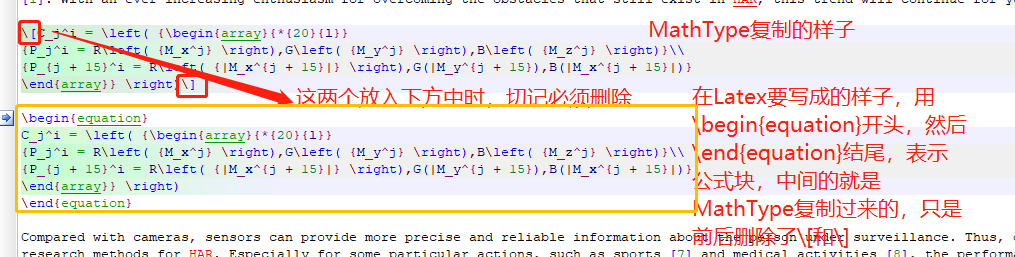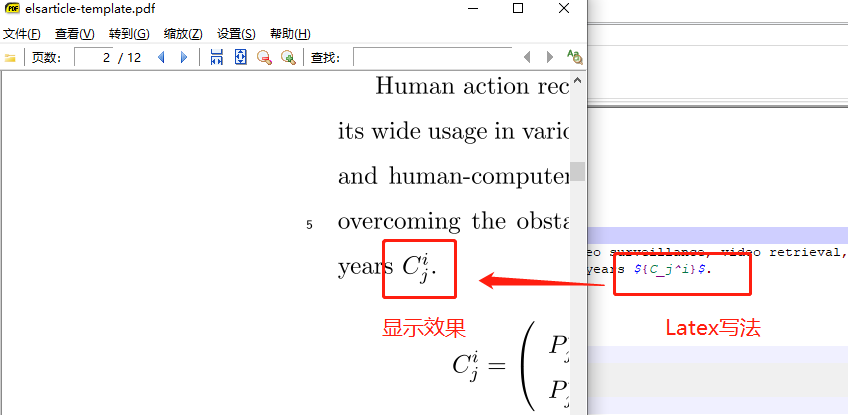### 2.4 表的制作

Latex中第二个难搞的是表格制作，这个真的是，前面觉得公式有点复杂，其实看完就是设置下MathType后，复制到begin/end里删除就完了，但是表格，真的复杂，为什么呢，表格大家都知道是变化无穷的，比如合并居中，三行五列等等，每个人的表格都不相同，第一次弄，这个也啃了好久，最后找到了点通用方法，可以快一点，分享如下：

### 2.4.1 表格制作，

\usepackage{booktabs}
\usepackage{threeparttable} %三线栏包，其实可以画成任何一行你想要画线分割的行处
\usepackage{multirow} %跨行跨列必用包
\usepackage{graphicx}

### 2.4.2 表格基本结构

\begin{table}[tp]   %[tp]控制浮动
\centering
\setlength\tabcolsep{1pt}   %控制列间距
\fontsize{6.5}{8}\selectfont %控制字体大小
\resizebox{\linewidth}{!}{   %表格超出会缩放页面合适大小
... 这部分就是实际表格部分
\end{table}

### 2.4.3 表格实际内容部分

（它可以支持你在本地写好的表格和数据，只支持CSV格式，然后导入这个工具中，然后我们在这个网站中去可视化的操作成我们想要的效果，和合并居中，加颜色加粗等，如下图，具体的操作和说明也见图中）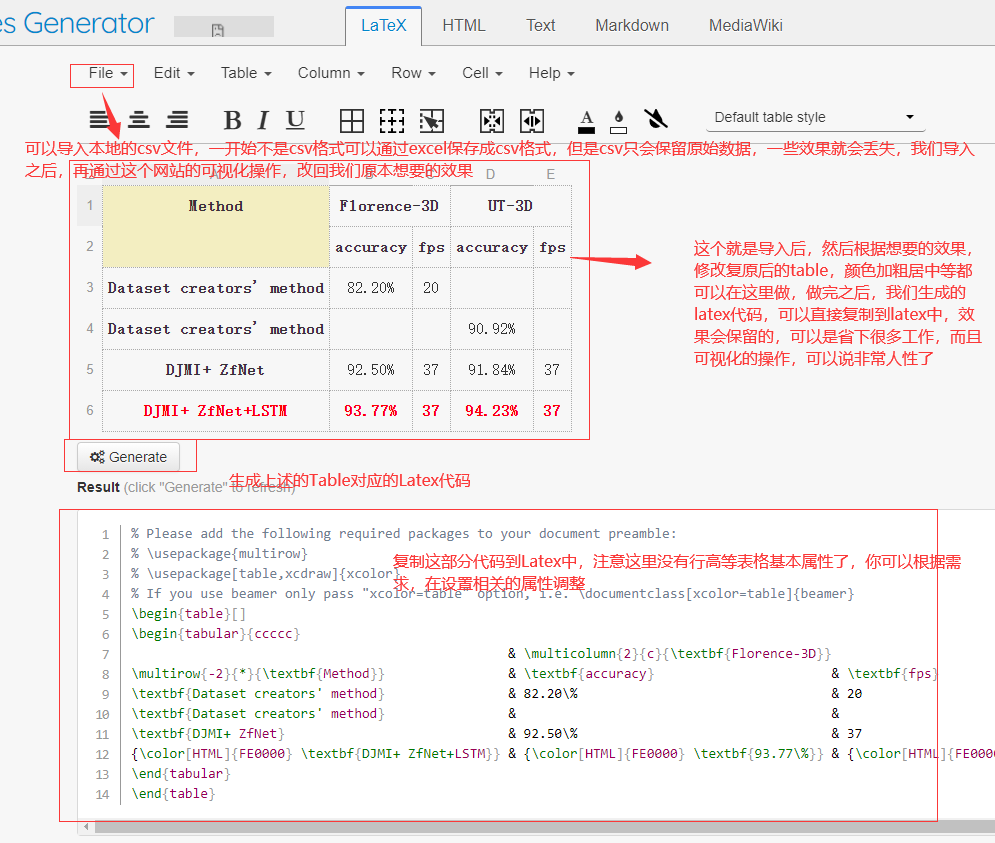(Tips：如果我们先设置了表格的属性，如行高等，使用了三线表等属性，那么我们只要上述图中\begin{tabular}开始到相应结束中的代码，一般也是只要这部分的内容，它才是我们通过神器生成的具体内容，至于前后的架子，还是用我们自己想要的，如下代码，使用了三线格，我只用了\begin{tabular}开始后对应生成具体表格内容)

\renewcommand{\arraystretch}{1} %控制行高
%\newcommand{\tabincell}{\begin{tabular}{@{}#1@{}}#2\end{tabular}}
\begin{table}[t]
\centering
\setlength\tabcolsep{1pt}
\fontsize{6.5}{8}\selectfont
\resizebox{\linewidth}{!}{
\begin{threeparttable}
\caption{这个就是表分名字 编号也是自己按顺序自动编的.}
%%\label{tab:performance_comparison}
\begin{tabular}{ccccccccc}   %%{p{1.5cm}p{1.6cm}p{1cm}p{1cm}p{1cm}p{1cm}p{1cm}p{1cm}p{1cm}}
\toprule
%%\bf{Data Set}&\bf{Score Item}&\bf{leNet-5}&\bf{AlexNet}&\bf{ZfNet}&\bf{DenseNet121}&\bf{VGG16}&\bf{VGG19}&\bf{ResNet50}\cr
%%\midrule
\textbf{Data Set}              & \textbf{Score Item}            & \textbf{leNet-5}               & \textbf{AlexNet}               & \textbf{ZfNet}
& \textbf{DenseNet121}           & \textbf{VGG16}                 & \textbf{VGG19}                 & \textbf{ResNet50}              \\
\midrule
\multirow{6}{*}{\textbf{Florence-3D}} & \textbf{\tabincell{c}{based on \\ original samples}}  & 79.07\%            & 74.42\%                        & 62.79\%
& 20.93\%                        & 76.74\%                        & 58.14\%                        & 37.21\%                        \\
& \textbf{\tabincell{c}{based on \\ generated samples}} & 83.47\%            & 90.80\%                        & 92.50\%
& 92.87\%                        & 87.95\%                        & 83.37\%                        & 88.80\%                        \\
& \textbf{\tabincell{c}{accuracy \\ improvement}}       & {\color[HTML]{FE0000} 4.40\%}  & {\color[HTML]{FE0000} 16.38\%} & {\color[HTML]{FE0000} 29.71\%} & {\color[HTML]{FE0000} 71.94\%} & {\color[HTML]{FE0000} 11.21\%} & {\color[HTML]{FE0000} 25.23\%} & {\color[HTML]{FE0000} 51.59\%} \\
\midrule
\multirow{6}{*}{\textbf{UT-3D}} & \textbf{\tabincell{c}{based on \\original samples}}  & 67.50\%                        & 67.50\%                        & 72.50\%                        & 50.00\%                        & 65.00\%                        & 37.50\%                        & 47.50\%                        \\
& \textbf{\tabincell{c}{based on \\ generated samples}} & 82.36\%                        & 85.87\%                        & 91.84\%                        & 88.71\%                        & 85.44\%                        & 83.09\%                        & 94.87\%                        \\
& \textbf{\tabincell{c}{accuracy \\ improvement}}       & {\color[HTML]{FE0000} 14.86\%} & {\color[HTML]{FE0000} 18.37\%} & {\color[HTML]{FE0000} 19.34\%} & {\color[HTML]{FE0000} 38.71\%} & {\color[HTML]{FE0000} 20.44\%} & {\color[HTML]{FE0000} 45.59\%} & {\color[HTML]{FE0000} 47.37\%} \\
\bottomrule
\end{tabular}
\end{threeparttable}
}
\end{table}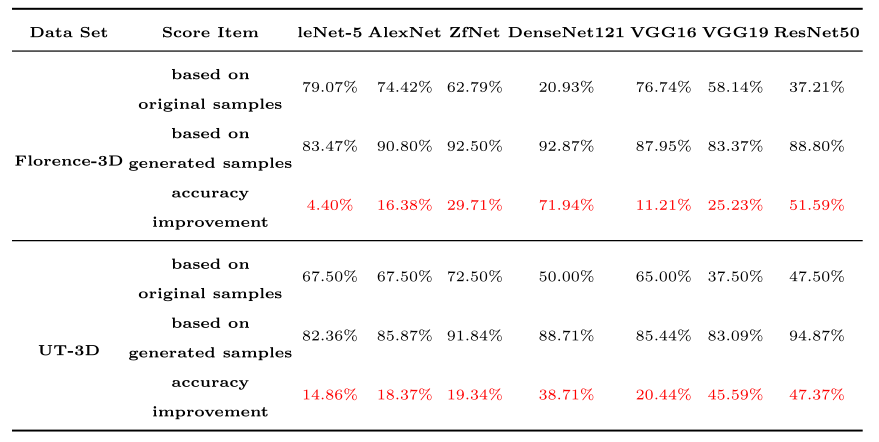Tips；三线格表格，要用到包，即上面说的导包，像三线表格的框架，不含具体内容，其实这样的

\renewcommand{\arraystretch}{1} %控制行高
%\newcommand{\tabincell}{\begin{tabular}{@{}#1@{}}#2\end{tabular}}
\begin{table}[t]
\centering
\setlength\tabcolsep{1pt}
\fontsize{6.5}{8}\selectfont
\resizebox{\linewidth}{!}{
\begin{threeparttable}
\caption{表格名字.}
... 这部分的内容，我是直接复制那个网站设置好并生成的相应\begin{tabular}的内容
\end{threeparttable}
}
\end{table}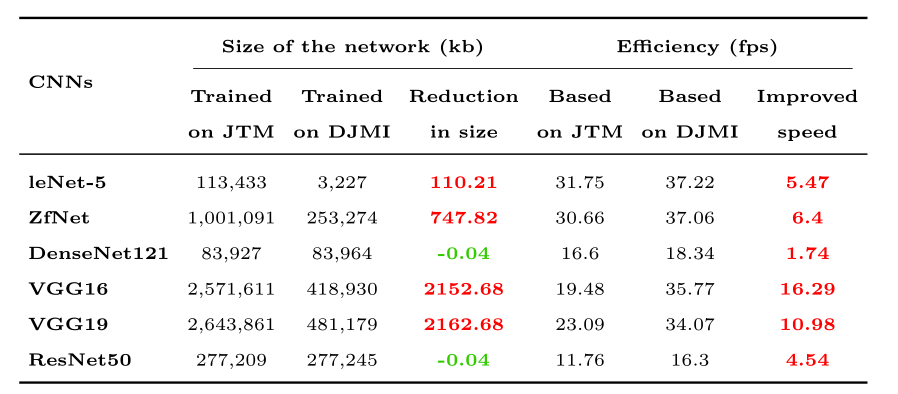\renewcommand{\arraystretch}{1} %控制行高
%\newcommand{\tabincell}{\begin{tabular}{@{}#1@{}}#2\end{tabular}}
\begin{table}[t]
\centering
\setlength\tabcolsep{3pt}
\fontsize{6.5}{8}\selectfont
\resizebox{\linewidth}{!}{
\begin{threeparttable}
\caption{表格名字.}
%%\label{tab:performance_comparison}
\begin{tabular}{lcccccc}
\toprule
\multirow{3}{*}{\textbf{CNNs}} & \multicolumn{3}{c}{\textbf{Size of the network (kb)}}   & \multicolumn{3}{c}{\textbf{Efficiency (fps)}}                                          \\
\cmidrule(lr){2-7}
& \textbf{\tabincell{c}{Trained \\ on JTM}} & \textbf{\tabincell{c}{Trained \\ on DJMI}} & \textbf{\tabincell{c}{Reduction \\ in size}} & \textbf{\tabincell{c}{Based \\ on JTM}} & \textbf{\tabincell{c}{Based \\ on DJMI}} & \textbf{\tabincell{c}{Improved \\ speed}}               \\
\midrule
\textbf{leNet-5}                & 113,433                 & 3,227                    & {\color[HTML]{FE0000} \textbf{110.21}}  & 31.75                 & 37.22                  & {\color[HTML]{FE0000} \textbf{5.47}}  \\
\textbf{ZfNet}                  & 1,001,091               & 253,274                  & {\color[HTML]{FE0000} \textbf{747.82}}  & 30.66                 & 37.06                  & {\color[HTML]{FE0000} \textbf{6.4}}   \\
\textbf{DenseNet121}            & 83,927                  & 83,964                   & {\color[HTML]{32CB00} \textbf{-0.04}}   & 16.6                  & 18.34                  & {\color[HTML]{FE0000} \textbf{1.74}}  \\
\textbf{VGG16}                  & 2,571,611               & 418,930                  & {\color[HTML]{FE0000} \textbf{2152.68}} & 19.48                 & 35.77                  & {\color[HTML]{FE0000} \textbf{16.29}} \\
\textbf{VGG19}                  & 2,643,861               & 481,179                  & {\color[HTML]{FE0000} \textbf{2162.68}} & 23.09                 & 34.07                  & {\color[HTML]{FE0000} \textbf{10.98}} \\
\textbf{ResNet50}               & 277,209                 & 277,245                  & {\color[HTML]{32CB00} \textbf{-0.04}}   & 11.76                 & 16.3                   & {\color[HTML]{FE0000} \textbf{4.54}} \\
\bottomrule
\end{tabular}
\end{threeparttable}
}
\end{table}

Tips:反正图的制作，基本就是那样，要善于利用神器网站，减低工作量和复杂度。

### 2.5 图的制作

\begin{figure}[t]  %%t表示放在页面顶部
\setlength{\abovecaptionskip}{-0.3in}
\setlength{\belowcaptionskip}{-0.1in}
\centering
\includegraphics[width=4.8in]{fig6pdf图片存放的位置，是相对位置，建议和编辑的latex文件放同一目录下.pdf}
\caption{图片的名字. }
\end{figure}

### 2.6 参考文献

\section*{References}
\begin{thebibliography}{99}
\bibitem{ref1索引这个到时候是要在文中具体引用用的}A. Bakar, A.R. Syed, Advances in human action recognition: an updated survey, IET Image Processing. 13 (13) (2019) 2381-2394.
\bibitem{ref2}C. Rougier, J. Meunier, A.S. Arnaud, J. Rousseau, 3D head tracking for fall detection using a single calibrated camera, Image and Vision Computing. 31 (3) (2013) 246-254.
\bibitem{ref3}S. Gasparrini, E. Cippitelli, S. Spinsante, E. Gambi, A depth-based fall
\end{thebibliography}

and human-computer interaction \cite{ref1}. With an ever-increasing enthusiasm for overcoming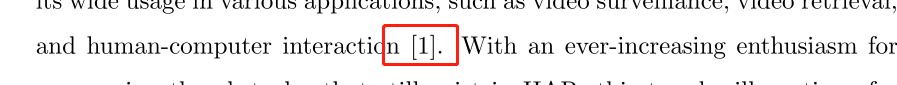### 致谢参考:09-26
08-05
01-295万+
10-245298
01-202312
05-265202
11-24
07-051万+